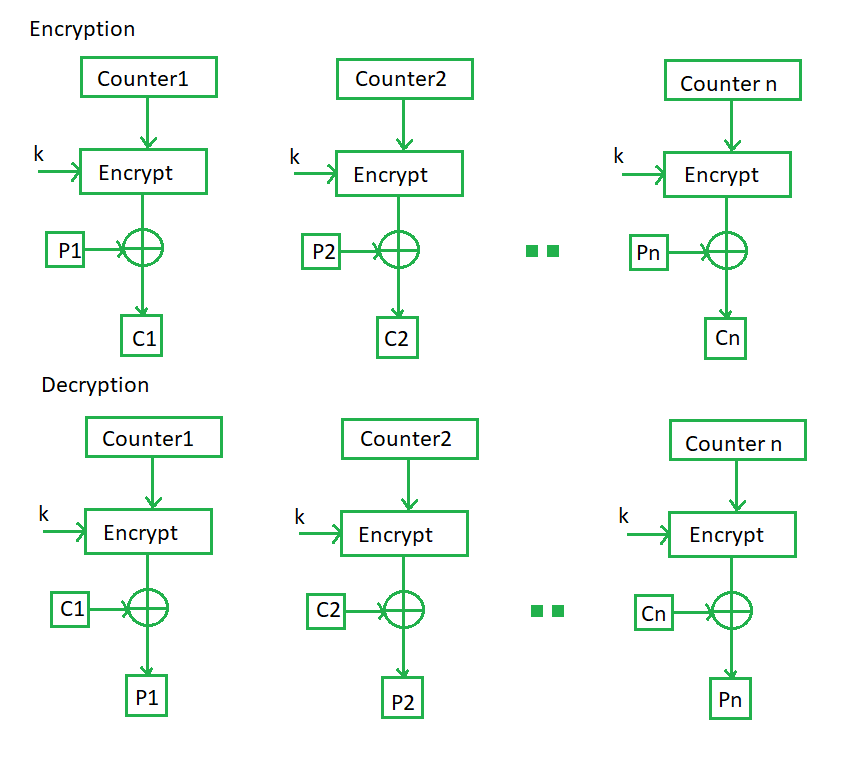Open in App
Not now

# Block Cipher modes of Operation

• Difficulty Level : Easy
• Last Updated : 22 Nov, 2022

Encryption algorithms are divided into two categories based on the input type, as a block cipher and stream cipher. Block cipher is an encryption algorithm that takes a fixed size of input say b bits and produces a ciphertext of b bits again. If the input is larger than b bits it can be divided further. For different applications and uses, there are several modes of operations for a block cipher.

Electronic Code Book (ECB) –
Electronic code book is the easiest block cipher mode of functioning. It is easier because of direct encryption of each block of input plaintext and output is in form of blocks of encrypted ciphertext. Generally, if a message is larger than b bits in size, it can be broken down into a bunch of blocks and the procedure is repeated.

Procedure of ECB is illustrated below: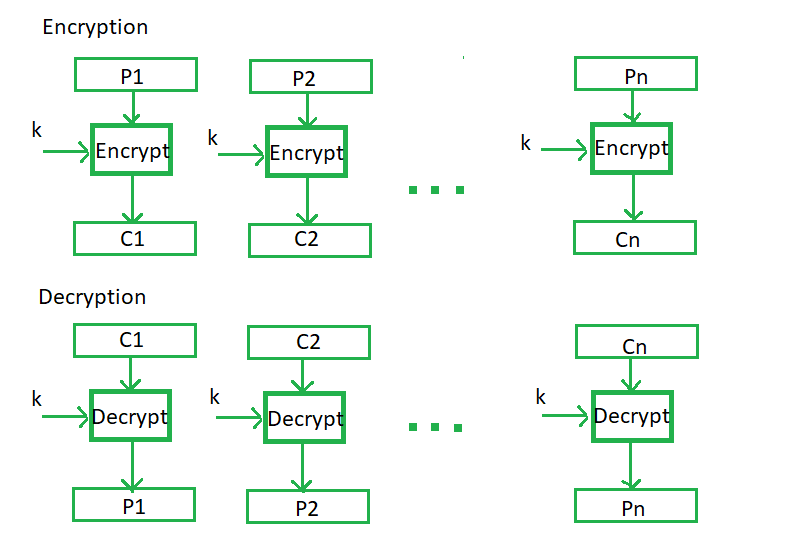• Parallel encryption of blocks of bits is possible, thus it is a faster way of encryption.
• Simple way of the block cipher.

• Prone to cryptanalysis since there is a direct relationship between plaintext and ciphertext.

Cipher Block Chaining –
Cipher block chaining or CBC is an advancement made on ECB since ECB compromises some security requirements. In CBC, the previous cipher block is given as input to the next encryption algorithm after XOR with the original plaintext block. In a nutshell here, a cipher block is produced by encrypting an XOR output of the previous cipher block and present plaintext block.

The process is illustrated here: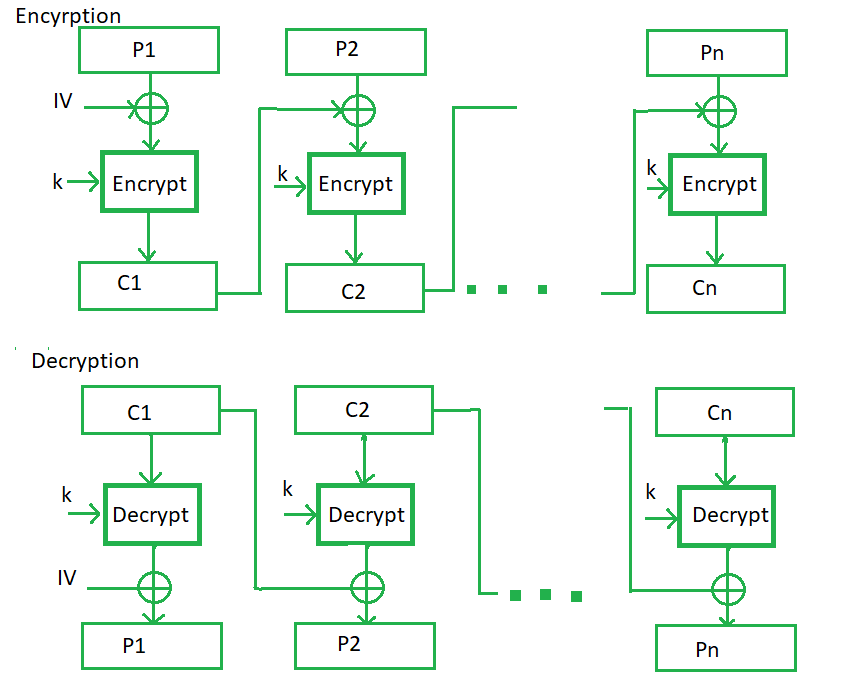• CBC works well for input greater than b bits.
• CBC is a good authentication mechanism.
• Better resistive nature towards cryptanalysis than ECB.

• Parallel encryption is not possible since every encryption requires a previous cipher.

Cipher Feedback Mode (CFB) –
In this mode the cipher is given as feedback to the next block of encryption with some new specifications: first, an initial vector IV is used for first encryption and output bits are divided as a set of s and b-s bits.The left-hand side s bits are selected along with plaintext bits to which an XOR operation is applied. The result is given as input to a shift register having b-s bits to lhs,s bits to rhs and the process continues. The encryption and decryption process for the same is shown below, both of them use encryption algorithms.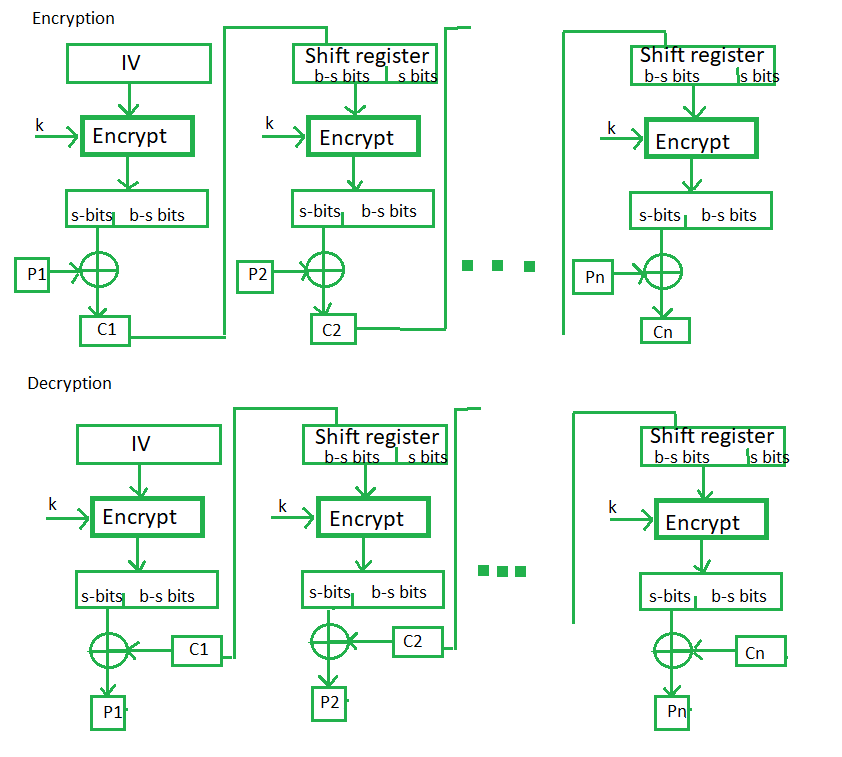• Since, there is some data loss due to the use of shift register, thus it is difficult for applying cryptanalysis.

• The drawbacks of CFB are the same as those of CBC mode. Both block losses and concurrent encryption of several blocks are not supported by the encryption. Decryption, however, is parallelizable and loss-tolerant.

Output Feedback Mode –
The output feedback mode follows nearly the same process as the Cipher Feedback mode except that it sends the encrypted output as feedback instead of the actual cipher which is XOR output. In this output feedback mode, all bits of the block are sent instead of sending selected s bits. The Output Feedback mode of block cipher holds great resistance towards bit transmission errors. It also decreases the dependency or relationship of the cipher on the plaintext.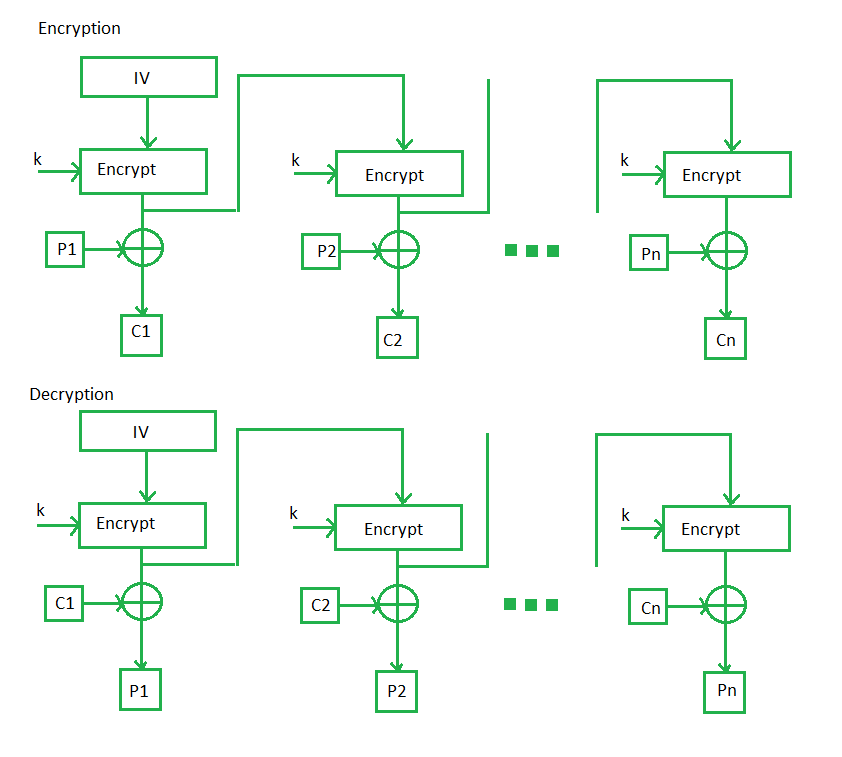• In the case of CFB, a single bit error in a block is propagated to all subsequent blocks. This problem is solved by OFB as it is free from bit errors in the plaintext block.

• The drawback of OFB is that, because to its operational modes, it is more susceptible to a message stream modification attack than CFB.

Counter Mode –
The Counter Mode or CTR is a simple counter-based block cipher implementation. Every time a counter-initiated value is encrypted and given as input to XOR with plaintext which results in ciphertext block. The CTR mode is independent of feedback use and thus can be implemented in parallel.

Its simple implementation is shown below: